## Thursday, December 23, 2010

### Math and Physics: Creative Arts?

My favorite approach towards math is through drawing geometric figures like circles, triangles, squares... They link the mathematical abstraction to the physical reality, whatever that may mean. They help to visualize patterns that may remain hidden when described with symbols. Doodling geometric figures allow digressive exploration of otherwise marked out paths and thus favor creative and curiosity-driven approaches towards math. Although I am not a mathematician, I practice math for my job and as a hobby. I subscribe to views expressed by G.H. Hardy in his Mathematician's Apology
I am interested in mathematics only as a creative art.
or by Paul Halmos in his Mathematics as a Creative Art
Mathematics is far closer to an art than it is to the business of equation-solving.
or by Paul Lockhart in his Mathematician's Lament
I’m just playing. That’s what math  is— wondering, playing, amusing yourself with your imagination.
Interestingly, in these quotes, math could be replaced by physics. Concerning me, it would likewise express my interest in physics. What if all of math and physics could be expressed through art? Well, it should.

## Sunday, November 28, 2010

### Geometric representations of higher degree binomials

A binomial is a mathematical expression with two terms, say x and y. The simplest binomial is the sum of those two terms x+y. Powers of this binomial (x+y)n are ruled by the so-called binomial theorem, which states that the coefficients of the expansion terms xpyq are given by the Pascal triangle entries. So we have:
(x+y)0 = 1
(x+y)1 = 1.x + 1.y
(x+y)2 = 1.x2+ 2.xy + 1.y2
(x+y)3 = 1.x33.x2y + 3.xy2 + 1.y3
(x+y)4 = 1.x44.x3y + 6.x2y2 + 4.xy3 + 1.y4
and so forth ...

But where do these coefficients come from? You can just be satisfied by the result algebraic calculation, or you could look for geometric representations. For instance, a nice geometric explanation is given at the www.mathaware.org site.Geometric proof of square binomial (original at The Geometry of the Binomial Theorem, Math Awareness Month site)Geometric proof of cubic binomial (original at The Geometry of the Binomial Theorem, Math Awareness Month site)
For the second degree binomial, the Pascal triangle entries emerge naturally as the number of squares or rectangles with area given by the expansion terms xpyq. For third degree binomials, they emerge as the number of cubes or parallelepipeds with volume given by the expansion terms. And for higher degree binomials, they emerge as the number of hypercubes and hyperparallelepipeds given by the expansion terms, so an Euclidean geometric representation for those higher degree binomials seems impossible.

This suggested impossibility prompted me to have a closer look at geometric representations of binomial expansions. As a matter of fact a number to the fourth power x4 is just a number, but it is also a square x4 = (x2)2. And there is no impossibility in representing numbers and squares geometrically, so the suggested impossibility is only an impossibility along the common line of thought, which sees cubic expansions as volumes. Along another line of thought, which seems to have been unnoticed, cubic expansions can be seen as areas, and then geometric representations of higher degree binomials become possible. The following figures illustrate this fact for the special case where x+y is normalized to 1 (for arbitrary x+y, one just has to rescale the figure each time, the pattern remains the same).

For the binomial (x+y)1 = 1.x + 1.y, we can divide a unit square into two rectangles, one rectangle of area x and one rectangle of area y, see Figure 1.
For the binomial (x+y)2 = 1.x22.xy + 1.y2, we can divide the unit square into two squares, one of area x2 and one of area y2, plus two rectangles of area xy, see Figure 2.
For the binomial (x+y)3 = 1.x33.x2y + 3.xy2 + 1.y3, we can divide each of the previous squares and rectangles into proportions x and y, giving eight rectangles, one of area x3one of area y3, plus three rectangles of area x2y and three rectangles of area xy2, see Figure 3.

For the binomial (x+y)4 = 1.x44.x3y + 6.x2y2 + 4.xy3 + 1.y4, we can again divide each of the previous squares and rectangles into proportions x and y, giving one square of area x4, one of area y4, four squares and two rectangles (giving six) of area x2y2, four rectangles of area x3y and four rectangles of area xy3, see Figure 4.

And we could go further indefinitely, doodling areas of incrementing powers, just in 2D, without any reference to unintuitive hyperspaces.

## Sunday, October 24, 2010

### Explaining electron spin and Pauli exclusion principle to children

Fundamental particles are the building blocks of nature, of which the photon and the electron have the most visible impact on our everyday life. Photons are all pervasive. If they have the right energy, they can stimulate your eyes' photoreceptor cells. At other energies they will warm you up because they radiate from a warm object. Electrons are more energetic than the photons. They can either be free in space, or bound in atoms. Through their motion, they transmit motion to photons, which in turn can excite other electrons at distant places. This phenomenon, known as electromagnetism, is used in all wireless transmissions. Photons are the electromagnetic force carriers and electrons are the electromagnetic force sources.

In order to understand the behavior of photons and electrons, it is important to have analogies that help us keeping track of them. In previous posts, I mentioned some helpful analogies for photons (for example at this post on polarization). Although electrons also show wave behavior, they act a bit differently from photons. You can not stack electrons near to one another, except if they have compatible spinning motions. For spinning motions to be compatible means that the electrons must:
• either spin at rates whose proportions are expressed with integers: for example one electron spins twice as fast as the other electron,
• or spin in different directions, if they spin with the same velocity.I sometimes come across situations that remind me of electrons. If you're standing in the bus or in the metro, you grip a pole to keep equilibrium. In the metro-train in my Paris suburb, the poles occur in pairs, like in the picture aside. When my children were younger, one of their favorite games was to spin around those poles. For parents, if you let your kids spin around the poles disorderedly, this game can be quite stressful, ending with fighting or crying. I used to explain to them that they had to spin like electrons in atoms. If one kid spins in one direction, the other kid needs to spin in opposite direction, in order to avoid hard clashes. I recently asked them if they could do it again so that I could put it on movie and post it to illustrate this electron analogy. But they've grown up and are now ashamed to play such games:-) So I decided to create the following simple animations that illustrate the electron spin and the Pauli exclusion principle.A kid spinning around the pole is alike an electron spinning around a proton in its state of minimum energy, see Figure 1. Physicists designate the spinning direction with the help of the right hand rule. The kid of Figure 1 therefore has its spin down.

If your second kid spins in the same direction around the other pole, you can be sure that this game won't last for long. Their motions are incompatible and it ends up with a clash, see Figure 2.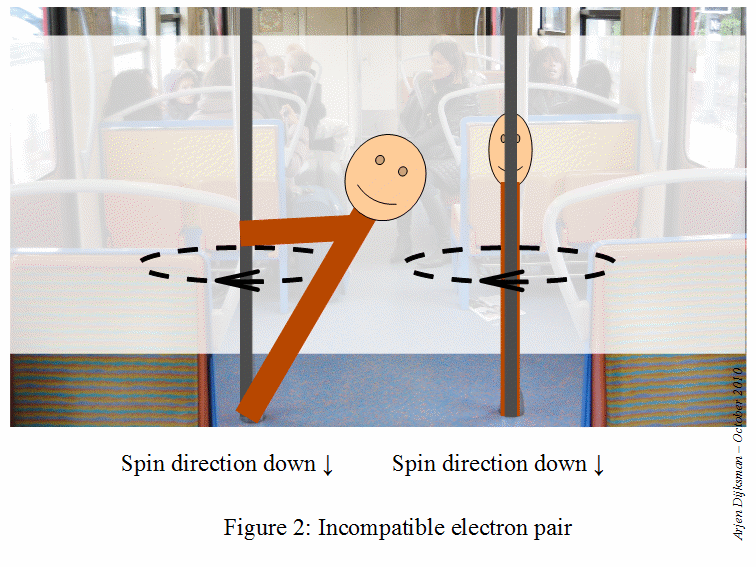If you want them to play peacefully, you need to instruct them to follow a natural rule: the Pauli exclusion principle, illustrated in Figure 3. Electrons with same spinning velocity and sharing the same space can only occur if their spins are opposite. Very useful rule to keep harmony in the family!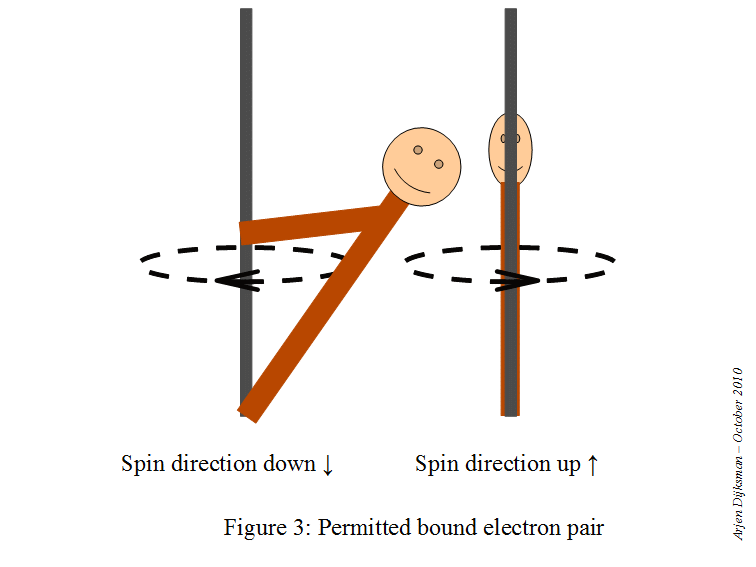## Thursday, October 21, 2010

### Volume of a bead vs. volume of a sphere

Imagine you drill a hole through the center of a sphere. The remaining object (a bead, if we think of a little pearl with a hole) has an interesting property as pointed out by Pat Ballew on his blog at the end of this post. Whatever the radius of the initial sphere, the volume of the bead depends only on its height h (see following figure taken from Pat's blog). More precisely, its volume is the same as the volume of a sphere with diameter h. A proof of this property has been given by Pat in a later post.As I was thinking about it, I thought about another way to prove it, inspired by Mamikon's visual calculus method.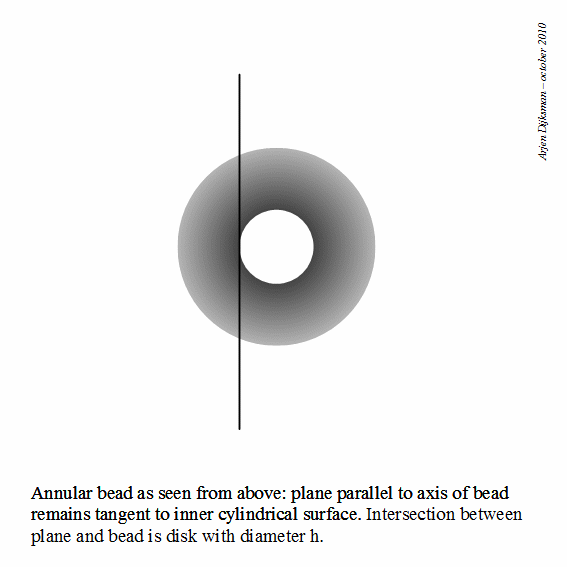If we think of a plane parallel to the axis of the bead and tangent to its inner cylindrical surface, the intersection of the bead and the plane is a disk of diameter h. If we now rotate the plane around the axis for a whole turn such that it remains tangent to the inner surface, the disk will also rotate a whole turn, as if it sweeps the volume of a sphere of diameter h.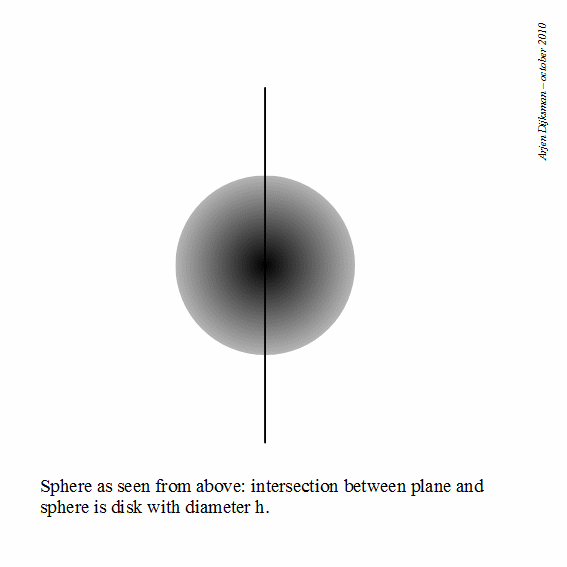Post scriptum. Afterwards I found a drawing of such a bead in Mamikon's original notes (see second page of his drawings).

## Sunday, October 17, 2010

### In memoriam: Georges Charpak and Maurice Allais

Time goes by and people die. And so do great scientists. There is Benoit Mandelbrot (November 20, 1924 – October 14, 2010) who developed the study of fractals, because he "decided to go into fields where mathematicians would never go because problems were badly stated". Two others, less known in the English speaking world and who are an example to me have also left us recently. I name: Georges Charpak and Maurice Allais.Georges Charpak (March 8, 1924 – September 29, 2010) was born in the little town Dubrovytsia located in a region where the political and social situation was very complicated at that time. The region was essentially populated with Ukrainian and Yiddish speaking people. It had suffered the post-WW1 Polish-Russian war and belonged to Poland at the time of his birth. Charpak's family had the opportunity to flee to France, which saved him from later WW2 exterminations of Jews in his natal country. In France, the situation was much better, Georges calling it even "paradise". During the 1920-30s, there was a tolerant spirit in France, allowing him to make friends with people of all origin. The Nazi occupation of France during WW2 brought new dangers for him. He had to change his name to George Charpentier, entered the French Resistance, he was imprisoned, participated to mutiny in the prison, escaped the punishment fusillade for the mutineers (he heard the ball flying around his ears). He was deported to the concentration camp of Dachau and was saved from extermination again because the Nazis could use his young guy's force in Dachau instead of sending him to more severe camps. His career as an experimental physicist started after the war with a thesis on particle detectors in Frédéric Joliot-Curie's group. He excelled in building simple detectors. His wire-detectors slightly replaced the historical bubble and ionization-chambers. He further worked at CERN and one of his detectors, the multi wire proportional chamber ("not the most elegant" in his words), earned him the Nobel in 1992. He also lectured at the ESPCI, where I'm currently PhD student. Apart from this exceptional course, after his Nobel, he had the nobility of mind to start a hands-on program for elementary school students "La Main à la Pâte" (literally Hand in the dough). I am fond of such initiatives because it brings experimental physics nearer to us. It is always preferable to first discover by ourselves how Nature works before learning how to formulate its laws through math. Too often, we learn the formula of a physical law before having experimented it personally.
"If there's one thing to do, it's to engage in education." ~ Georges Charpak.Maurice Allais (May 31, 1911 – October 9, 2010) was born earlier, before WW1. His father died in a German prison during WW1. Early loss of his father left a profound mark on the rest of his life. He devoted his life to the comprehension of all things he encountered. His passions were history, science, economics, physics. He excelled in all disciplines during his education. He had the opportunity to visit the United States in 1933 and was so impressed by the Great Depression and the inability to solve the crisis, that he studied by himself the principles that would secure economic wealth. The life-long product of this work earned him the Nobel Economics in 1988. I'm not a specialist in Economics, but as far as I understand, one of his findings (before other economists) is the Golden rule of savings rate, which states that the rate of interest a banker applies should be equal to the rate of economic growth: an equilibrium law applied to economics. At the beginning of his professional career, Maurice Allais taught economics at the Ecole des Mines. I suppose Georges Charpak, student at that same school, must have followed some of his lectures (*footnote). While Georges Charpak engaged in "normal" physics, Maurice Allais pondered over the foundations of physics. He wasn't satisfied about the interpretation of relativity and quantum theories. As a physicist, he needed to find it out for himself. In the 19th century tradition, against mainstream, he began to conduct experiments on a pendulum of his invention in order to investigate periodical fluctuations in gravity and electromagnetism and their influence by planetary motion. The interesting thing is that he found unexplained effects, among which the most famous is the "Allais effect", a deviation of the oscillatory plane of the pendulum during solar eclipses. Maurice Allais published some books in French where he details the results of his investigations. These effects remain unexplained today, likewise the Pioneer anomaly. I have no settled idea about these effects. I think that such effects suffer from capricious cosmological (photon, graviton, muon or whatever other particles) weather. One can find some seasonal regularities though. Further investigation is left to us, curious experimenters, satisfied only by what Nature teaches us.

*Update November 20, 2010: This was confirmed to me by close relatives to Maurice Allais and Georges Charpak.

Credit of the portraits of both Nobel Prize winners by Studio Harcourt Paris.

## Wednesday, October 13, 2010

### Follow-up of my FQXi essay: Ordinary analogues for Quantum Mechanics

Today I had the good surprise to discover the article "Quantum mechanics writ large" written by John W. M. Bush, Professor of Applied Mathematics at MIT, promoting the work of Couder, Fort et al. on the bouncing droplets. John Bush writes: "At the time that pilot wave theory was developed and then overtaken by the Copenhagen interpretation as the standard view of quantum mechanics, there was no macroscopic pilot wave analog to draw upon. Now there is."

I'm totally in line with this opinion. Quantum mechanics has macroscopic analogues which have so far nearly never been discussed and from which we would learn a lot. There has already been some discussion along with my 2009 FQXi essay. In the abstract, I wrote something similar to John Bush: "Classical physics was not sufficiently advanced to deal with macroscopic particle-wave systems at the birth of quantum mechanics. Physicists therefore lacked references to compare quantum with analogous macroscopic behaviour. After consideration of some recent experiments with droplets steered by waves, we examine possibilities to give some intuitive meaning to the rules governing the quantum world."

So, I hope this new article will gain much attention and foster discussion about macroscopic analogues for quantum behavior.

## Saturday, August 28, 2010

### Alternative Pythagorean quadruples and other extensions to Pythagoras theorem

The vertices of an arbitrary triangle can be disposed onto two concentric circles such that the base is the diameter of the first circle (which I call the base circle) and the opposite vertex is on the second circle (which I call the leg circle). As there are three bases, there are generally three ways to arrange this setting. I mentioned in my preceding post that we can apply the Pythagorean-like relation a²+b²=c²±2t² to this triangle, where c is its base, a and b are the legs and t is the tangent to the inner circle emanating from the outer circle. For clarity, I reproduce an illustrative figure from my preceding post, for the case where the inner circle is the base circle.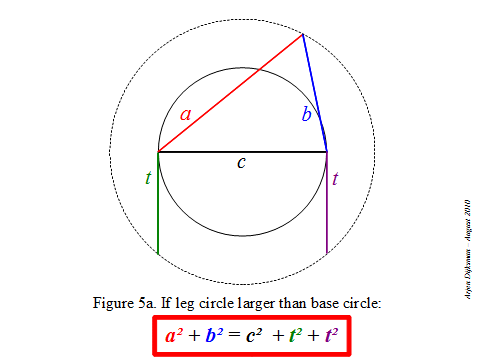Now, like for the classical Pythagoras relation, there are many features that can be investigated regarding this alternative relation. For example, I would like to better understand the relation of this 2D formula with the Cartesian 3D variant of Pythagoras: x²+y²+z²=d², which is analogous to the case where the leg circle is smaller than the base circle (see Figure 5c of preceding post). Another interesting aspect is the fact that the square of the tangent (multiplied by π) determines the area of the annular ring delimited by the base and the leg circles, as stated by Mamikon Mnatsakanian. This fact offers possibilities for areal representations of the squares or circles related to the sides of the arbitrary triangle, like we are acquainted to do with the squares related to the sides of a right triangle. Also, what would be interesting to develop is its relation with the law of cosines (as noticed by Pat Ballew) and the angle subtended by sides a and b. I guess this will leave enough stuff for future posts or conversations, or even papers in specialized journals.

As a conclusion, let me mention Pythagorean numerological features which provide stuff for entertaining puzzles. Maybe you know the Pythagorean triples, those sets of integer numbers (n,m,l) that verify n²+m²=l² and of which (3,4,5) is the simplest instance. The 3D Pythagoras relation allows an extension to Pythagorean quadruples, sets of integer numbers (n,m,l,k) that satisfy n²+m²+l²=k² and of which (1,2,2,3) is the simplest instance. I couldn't resist to look for some integer quadruples that satisfy a²+b²=c²+2t². Among them I found two nice quadruples with successive a,b,c :

(7,8,9,4) for which 7² + 8² = 9² + 2 × 4² = 113

(35,36,37,24) for which 35² + 36² = 37² + 2 × 24² = 2521

## Sunday, August 15, 2010

### A Pythagorean relation for any triangle?

Physics makes extensive use of the Pythagorean law relating the squares of the sides of a right triangle. The well-known a² + b² = c² relation is of special interest for the determination of distances and lengths of vectors, but also for energy conservation laws and Lorentz transformations. There are various relations that resemble the Pythagoras law, but none of them seems to have the usefulness of Pythagoras’ original one, as well as the “beauty” originating from its sole quadratic terms. When the vertex opposite to the hypotenuse runs on the circle determined by the right triangle, we have the Pythagoras relation illustrated in Figure 1.
Some time ago, I was made aware of another interesting Pythagorean law, discovered by Nguyen Tan Tai and which I mentioned in a previous post. For any triangle with sides a, b and c, if the vertex opposite to the base (say c) runs on any fixed circle centered at the center of c, we have the relation:

a² + b² = c² + constant

In order to have a better understanding of this quadratic relation, I tried to re-derive it in my own mental representation. I will call the fixed circle with the running vertex C the “leg circle”, because it is determined by the vertex that is common to legs AC and BC of the triangle (see Figure 2).When the leg circle is larger than the base circle, the legs AC and BC intersect the base circle and we can decompose the arbitrary triangle into two right triangles, for example as illustrated in Figure 3, the right triangles ABD and BCD.With the Pythagoras relation we than have:

AC² + BC² = (AD + CD)² + BC²
= (AD² + CD² + 2.AD.CD) + (BD² + CD²)
= AD² + BD² + 2.CD² + 2.AD.CD
= (AD² + BD²) + 2.(AD + CD).CD
= AB² + 2.AC.CD

But the product AC.CD is the power of point C with respect to the base circle, i.e. for every point C on the leg circle, AC.CD is constant and equal to the square of the tangent ray CT (see Figure 4).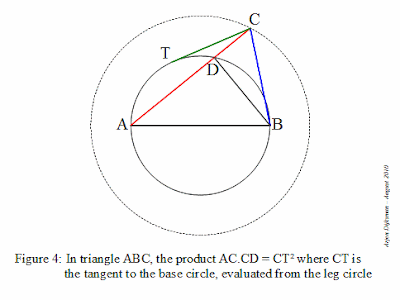If we use another notation, AC = a, BC = b, AB = c and CT = t, the relation becomes a nice equation with only quadratic terms:

a² + b² = c² + t² + t²

One can verify that if the leg circle has same size as the base circle, we retrieve the original Pythagoras relation :

a² + b² = c²

And if the leg circle is smaller than the base circle, we have:

a² + b² + t² + t² = c²

where t is now the tangent ray to the leg circle emanating from any point of the base circle.

We thus have a Pythagoras-like relation for any triangle given its base and leg circles, as illustrated by Figures 5a, 5b and 5c.## Sunday, June 6, 2010

### Lost theorem about angular proportions

Last week, I came across a so called missing theorem about angular proportions in a triangle, discovered (or rediscovered) by Leon Romain. This triangle construction is presented by user Linelites on youtube.

For such a "Romain triangle", the missing theorem states that, if one of the inner angles is twice another inner angle, one has the property a² = bc +c², where a, b and c are outlined in Figure 1.

The sine chord pattern presented in the Teaching sine function with spaghetti provides helpful insights for the missing theorem. In this pattern, all angles at the intersection of chords are integer multiples of a chosen unit angle or its complement, modulo 90°. All segment lengths in this pattern can therefore be written as sums or differences of cosine and sine products and ratios of that angle. Figure 2 pictures some measures of sine chords in a circle of unit diameter, for an arbitrary angle θ.

Figure 3 shows a Romain triangle for angle α in this pattern. There are numerous other Romain triangles in this pattern. Can you figure them out? With the help of the measures pictured in Figure 2, one can follow visually the elements of a proof for the missing theorem.

Side b is the sine of the chosen angle θ (Figure 4):

b = sinθ

Side c times sin equals b times sinθ (Figure 5):

c = sin²θ/sin

Side a equals b cosθ minus c cos (Figure 6)

a = sinθ cosθ - sin²θ cos /sin

Working out a² and bc +c², one finds that they are both equal to 2 sin²θ cosθ / sin3θ.

The interesting thing is that the sine chord pattern hosts plenty of "missing theorems" about angular proportions, which are only waiting to be (re)discovered.

______________________
Update (June 7, 2010): I corrected Figure 6, which held a wrong term.

## Sunday, April 11, 2010

### Teaching sine function with spaghetti

Sine functions appear everywhere in physics and mathematics. This seems to be related to the circular symmetry of space and to the periodic behavior of dynamical processes. The usual definition is that the sine of an angle is the ratio of the length of the opposite side to the length of the hypotenuse in a right triangle (definition at wikipedia). The sine is also often represented as the ordinate of a point running on a unit circle centered at the origin. Any right triangle can be put in that setting. The length of the blue segment on Figure 1 illustrates this definition for an arbitrary angle α, in a circle of unit radius.

It is of interest to have in mind other geometric representations of the sine function. This helps to associate figures with sine and cosine operations or identities. The red segment in Figure 1 shows such an alternative representation, because the sine is also a chord of a circle of unit diameter. When we increment the angle by steps α, one endpoint of the chord advances on the little circle, while the other endpoint remains fixed at the origin, as illustrated by the animation in Figure 2.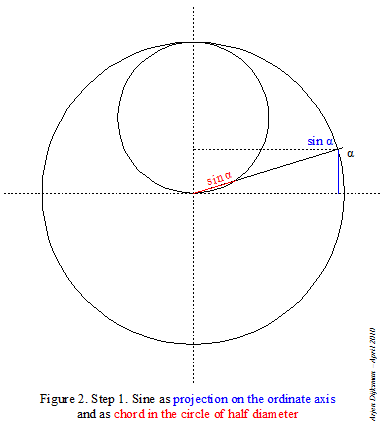When it represents the sine of angle α, the chord in the little circle spans an angle 2α. It is therefore a direct way to the angle multiplication and division by 2. Moreover, the endpoints of this chord can be placed two by two in any other direction on this circle. This enables one to find other variations on the recurrence pattern of the angle in the circle. During one of my circle drawing sessions, I was surprised by the stepwise zigzagging pattern of Figure 3.

Teaching the sine function with this zigzag pattern is particularly suited for daily life situations. Children like it, especially when you explain it at the table.## Monday, April 5, 2010

### Keeping track of the circle for integral representations of π

The fundamental constant π is characterized in many ways. Historically, it all began from tentative measurements of the circle's perimeter or area and gradually shifted into more advanced mathematics, in such a way that the link between the circle and modern characterizations of π faded away, see for example the formulas presented at Wolfram MathWorld or Wikipedia.

In the preceding posts, I mentioned infinite products as approximations for π. These may be seen geometrically as exhaustion methods, where the area of a polygon approaches the circular area alternately from above, from below, from above, from below, etc.
There are also integral representations of pi. In such integral representations, π appears in the quantitative value of the integral of a mathematical function. Visually, this is often represented as the area delimited by the bounds of the function. However, the relation with the circle is lost, when viewed under Cartesian coordinates. For example, the graph of the simplest instance of the Cauchy-Lorentz distribution, f(x)=1/(1+x²), "has nothing at all to do with circles or geometry in any obvious way" as quoted from last Pi-day Sunday function from Matt Springer's Built on Facts blog.In order to view the role of the circle in integral representations of π, we need to switch to alternative ways to visualize math functions. As an example, let's take the constant function y=f(x)=2. The function f maps an element x from a domain to the element y of the target. In this case, for every x, the target y has the constant value 2. With Cartesian coordinates, we are used to represent this function as a horizontal straight line, like in Figure 1a (click on the figure to view it enlarged). If however we write it as R=f(r)=2, where the function f maps any circle of radius r of the domain to a target circle of radius R=2, the same function can be viewed as a circle of constant radius, like in Figure 1b. So the same function f can be equally well viewed as a straight line or as a circle (x, y, r or R are only dummy variables).Now if we take another example, the linear function, y=f(x)=2x, we are often used to view it in Cartesian coordinates as a straight line with slope 2, like in Figure 1c. In the circular representation R=f(r)=2r, this works however differently. Because we are relating circles of the input domain to other circles of the target, for each circle of radius r, we need to draw the target circle of radius 2r. A single line won't do. For one value of r, we need to draw two circles. If we use blue circles for elements of the input domain and red circles for elements of the target, we could visualize it for successive values of r as an animation like in Figure 1d. In that way, we view the progression of the target circle as the input circle becomes larger.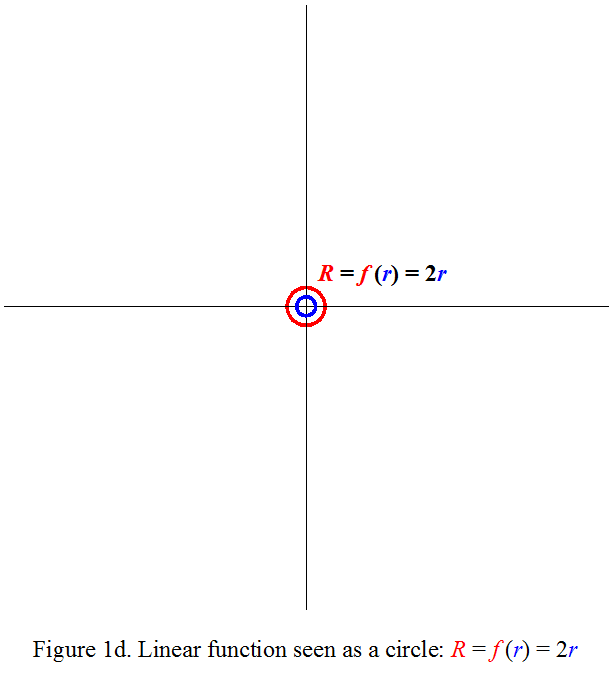Unlike the Cartesian representation which shows the progression of a function in a static graph, this circular representation needs a dynamic or recurrent process to get grip of the progression of the function. Therefore it isn't very adapted for illustrations in print media. On the other hand, it has the advantage of keeping track of the geometrical form of the circle. And that's exactly what we need in order to perceive the circular nature when π shows up in mathematical functions. The relation of the integral of the Cauchy-Lorentz distribution f(r)=1/(1+r²) with the circle can then be seen with the help of the geometric counterparts of arithmetic operations like addition, squaring and dividing. A convenient procedure is illustrated in the successive steps of Figure 2.

Step 1. Draw the input circle of radius r and the reference circle of radius unity.

Step 2. Determine r².Step 5. Find the target ring related to the input ring ranging over [r, r + dr]. This yields a ring of width dr/(1+r²). The location of this ring depends on the relative progression rates of r and r² (I've not yet found a straightforward explanation for this determination).Step 6. Integrate dr/(1+r²) for r running over all space. For r becoming larger and larger, the summed area tends towards the area of a circle of radius 1. For the positive half plane, this corresponds to the π/2 value found analytically.

The tricky step seems to be the way how to relate the progression between r and 1/(1+r²) in steps 5 and 6. One can verify for example the value of the integral at intermediate steps. For the integral from r=0 to 1, the value in the positive half plane must be π/4, which can be verified on the figure below.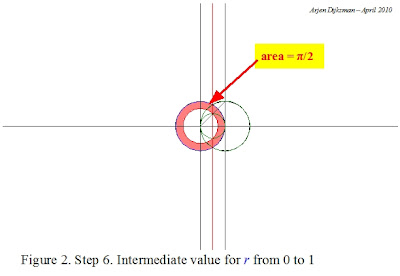In order to gain more insight on π, it could be of interest to develop skills for this circular representation.

## Sunday, March 28, 2010

### Wallis product for nested equilateral trianglesI'm discovering the Wallis product thanks to an approach involving geometry of areas. In order to relate that to what has already been written on the subject, here are some works which I would like to study in the coming time:
Maybe you have other suggestions on this subject.

Meanwhile, I tried to draw equilateral triangles in the exhaustion scheme of nested circles. We start from the concentric rings of same area as described in Figure 4 of the Variations of circular area division into equal parts post. Remember the inner circle has radius 1 and area π. Each subsequent Nth circle has radius square root of N (noted √N) and area Nπ. Then we can draw an equilateral triangle whose sides are tangent to the first circle. The neat thing about this procedure is that we can read out the pertaining numerical values right on the figure. Figure 1 shows us that the area of the equilateral triangle is 3√3. So the ratio between the outscribed equilateral triangle area and the circle area is equal to 3√3/π.

By drawing equilateral triangles tangent to circles of incrementing area Nπ, we discover the nested equal area division for equilateral triangles: the area between each equilateral triangle is equal to 3√3 (see Figure 2, you can click on the figure to view it enlarged). Among other interesting features, this figure contains the table of 4. For each triangle outscribing a circle of radius √N, its vertices are located on the circle of order 4×N.

If we outscribe alternately a circle, a triangle, a circle, a triangle, a circle, etc., the circles (and the vertices of the triangles) go through the subsequent powers of 4, see Figure 3. By changing the form of the triangle, we have access to other tables and powers. It seems there are plenty of properties that are hidden in this structure.
Now, we can try the Wallis exhaustion scheme on the nested circle and triangle structure. The procedure apparently goes something like:
Step 1. Draw the initial circle and triangle with area ratio 3√3/4π.
Step 2. A better fit would be to inscribe both figures in higher orders of the nested structure. But the area of the triangle is too small with respect to the circle. So we must enlarge the area of the triangle more than the circle. We enlarge the area of the triangle by 3 while leaving unchanged the area of the circle. This is illustrated in the figure below.
Step 3. But now the area of the triangle is too large with respect to the circular area. So for this fit, we must enlarge the area of the triangle a little less than the area of the circle. We therefore enlarge the area of the triangle by 3, while enlarging the area of the circle by 4, as illustrated below.
Step 4. But now the area of the triangle is too small with respect to the circular area. So for the next fit, we must enlarge the area of the triangle a little more than the area of the circle. We therefore enlarge the area of the circle by 6, while enlarging the area of the circle by 5, as illustrated below.
This exhaustion scheme that makes the area of equilateral triangles alternately larger, smaller, larger, smaller, etc... than the circular area can be written as:
This formula corresponds to an infinite product approximating π, which I've found in a French book Le fascinant nombre π by Jean-Paul Delahaye but I couldn't find it mentioned elsewhere. As approximation method, it is about twice as fast as Wallis' original product. This equilateral triangle exhaustion product is probably a special case in a large family of Wallis products, each of which pertaining to a particular geometrical configuration from which one tries to approximate π. The starting and recurrence conditions depend on the form and the initial area ratio between the polygon and the circle. The rules governing this exhaustion scheme in the general case still elude me.